# Experimental method for Bio-Data augmentation using only two observations for deep learning applications.

By Matthew Millar R&D Scientist at ユニファ

This blog will show a new experimental method for data augmentation geared towards bio-science for deep learning. This is important for several reasons. 1: Collecting data is time-consuming especially in collecting large enough observations for training deep learning models. 2: It can be difficult to collect or sample enough observations due to the lack of access or chances to make collections. 3: Collecting observations can only be done at certain times or during certain periods, or the period of time for sampling has passed so the collection of further/more observations are impossible. 4: There are few species available to collect samples from. These are just 4 simple reasons why data augmentation is needed for biological studies.

### Methods for Data Augmentation

The simplest method for data augmentation is to match the generated data both statistically and logically to the observed data. This means that the data that is generated should have a similar look and feel of the real-world data. The two data sets should have similar distributions, mean, modes, etc. to ensure that the data truly simulates the observed sequences. The simulated data should also be logically like the data that is observed. This means that the simulated data should not have outliers model into it as this will confuse any model. The augmented data should flow alongside the observations and almost mirror each observation. But, just copying the real observations is not an appropriate method for data augmentation. The observations should change slightly. For example, common methods for data augmentations in CNN are image rotation, flipping, cropping, changing color, etc. to create “new” unseen images for a CNN to be trained on. This is also true for numerical data, but not as easy as just flipping the numbers from 10 to 01 as they are not the same.

There are very few methods that exist for data augmentation for numerical data. There are even fewer geared specifically towards biodata or biostudies. This blog will show a new method for generating near-infinite observations based simply on the minimum and maximum observations in a data set.
The data set that I am using is a publicly available data set of Body Measurements (BDIMS)(Heinz, Peterson, Johnson, & Kerk, 2003). This data set is the girth and skeletal measurement of 247 men and 260 women.

Now let's get into the coding aspect of it:

### CODE

First, let's get all the import statements out of the way.

```import numpy as np
import pandas as pd
%matplotlib inline
import matplotlib.pyplot as plt
import pymc3 as pm
import theano
from statsmodels.formula.api import glm as glm_sm
import statsmodels.api as sm
from pandas.plotting import scatter_matrix
from random import randint
```

Next, we need to do some quick examination of the data we downloaded.

```# Read the data in from the csv file
print(data.columns)
```
```Index(['bia.di', 'bii.di', 'bit.di', 'che.de', 'che.di', 'elb.di', 'wri.di',
'kne.di', 'ank.di', 'sho.gi', 'che.gi', 'wai.gi', 'nav.gi', 'hip.gi',
'thi.gi', 'bic.gi', 'for.gi', 'kne.gi', 'cal.gi', 'ank.gi', 'wri.gi',
'age', 'wgt', 'hgt', 'sex'],
dtype='object')```

Now we know the colum names. Lets get rid of some of the data we dont want to make it simpler and easier to use.

```filter_data = data.filter(['sex','hgt','wgt', 'che.gi','hip.gi', 'kne.gi','thi.gi', 'ank.gi', 'wri.gi', 'wai.gi' ], axis=1)
```
```   sex    hgt   wgt  che.gi  hip.gi  kne.gi  thi.gi  ank.gi  wri.gi  wai.gi
0    1  174.0  65.6    89.5    93.5    34.5    51.5    23.5    16.5    71.5
1    1  175.3  71.8    97.0    94.8    36.5    51.5    24.5    17.0    79.0
2    1  193.5  80.7    97.5    95.0    37.0    57.3    21.9    16.9    83.2
3    1  186.5  72.6    97.0    94.0    37.0    53.0    23.0    16.6    77.8
4    1  187.2  78.8    97.5    98.5    37.7    55.4    24.4    18.0    80.0```

Much nicer. Now we only want to look at one subject as this is biological data. So we will filter out females from males and just look at males. This process will work on both sexes as the steps will be the same, but doing both at the same time will yield poor results as there are biological differences between males and females in general.

```# Split between male and female
# After sperating the two exes lets drop the sex collumn as we dont need it
male = male.drop(['sex'], axis=1)
male.describe()

hgt	wgt	che.gi	hip.gi	kne.gi	thi.gi	ank.gi	wri.gi	wai.gi
count	247.000000	247.000000	247.000000	247.000000	247.000000	247.000000	247.000000	247.000000	247.000000
mean	177.745344	78.144534	100.989879	97.763158	37.195547	56.497976	23.159109	17.190283	84.533198
std	7.183629	10.512890	7.209018	6.228043	2.272999	4.246667	1.729088	0.907997	8.782241
min	157.200000	53.900000	79.300000	81.500000	31.100000	46.800000	16.400000	14.600000	67.100000
25%	172.900000	70.950000	95.950000	93.250000	35.750000	53.700000	22.000000	16.500000	77.900000
50%	177.800000	77.300000	101.000000	97.400000	37.000000	56.000000	23.000000	17.100000	83.400000
75%	182.650000	85.500000	106.050000	101.550000	38.450000	59.150000	24.300000	17.850000	90.000000
max	198.100000	116.400000	118.700000	118.700000	45.700000	70.000000	29.300000	19.600000	113.200000
```

Now with the first step of preprocessing, we can get into the process of creating the dataset from only two points! These two points will be the minimum and maximum based on height. Height is chosen because this variable is the dominating variable in biology and bio-mass. Weight is normally heavily dependant on height (pun intended). The dependent variable will be weight. (X = height Y = weight).
So let's find the smallest and largest person in the dataset.

```# Find the smallest item based on height
# Create a new dataframe of the smallest and larget
min_max_male = pd.DataFrame(male[male.hgt == male.hgt.max()])
min_max_male = min_max_male.append(male[male.hgt == male.hgt.min()])
# Sort by height
sort_min_mix_male = min_max_male.sort_values('hgt')
print(sort_min_mix_male)
hgt   wgt  che.gi  hip.gi  kne.gi  thi.gi  ank.gi  wri.gi  wai.gi
105  157.2  58.4    91.6    91.3    35.5    55.0    20.8    16.4    80.6
126  198.1  85.5    96.9    94.9    39.2    54.4    27.5    17.9    82.5
ax1 = min_max_male.plot.scatter(x='hgt',y='wgt',c='DarkBlue')
```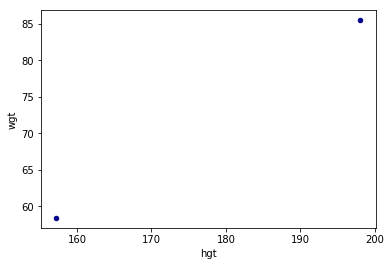Max Min PlotSo the first and simplest method to interpolation is linear regersion. This will give us a few extra points of missing data.

```# Now use linear regression to fill in some of the missing points
import numpy as np
from sklearn.linear_model import LinearRegression
x = np.array([min_max_male.hgt.min(),min_max_male.hgt.max()]).reshape((-1, 1))
y = np.array([min_max_male.wgt.min(), min_max_male.wgt.max()])
# Define a linear regerssion model
model = LinearRegression()
model.fit(x, y)
r_sq = model.score(x, y)
print('coefficient of determination:', r_sq)
print('intercept:', model.intercept_)
print('slope:', model.coef_)
```
```coefficient of determination: 1.0
intercept: -45.75941320293397
slope: [0.66259169]```

Now to make new points.

```prediction = []
gen_height = []
for i in range(int(min_max_male.hgt.min()), int(min_max_male.hgt.max())):
new_x = np.array(i).reshape((-1, 1))
gen_height.append(i)
pred = model.predict(new_x)
prediction.append(pred)

print(len(prediction))
print(len(gen_height))
print(prediction)
print(gen_height)
41
41
58.267481662591706
157
# Lets plot the results
import matplotlib.pyplot as plt

old_min_hgt = min_max_male.hgt.min()
old_max_hgt = min_max_male.hgt.max()
old_min_wgt = min_max_male.wgt.min()
old_max_wgt = min_max_male.wgt.max()

plt.plot(gen_height, prediction, 'ro')
plt.plot(old_min_hgt, old_min_wgt, 'bo')
plt.plot(old_max_hgt, old_max_wgt, 'bo')
plt.show()
```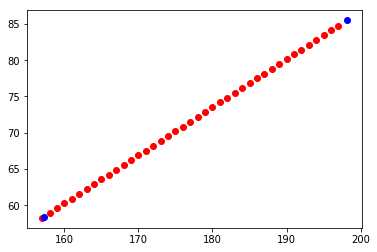Linear RegressionOk looks fine so far. The blue dots are the original data (min and max) and the red dots are the newly generated data. This makes sense as weight should increase as height increases. But, not really. There are variations in weight because of other factors. Also, 41 new points don't make a deep learning set.
Lets create a few more points:

```# Now lets fine tune the hieght veriable by a float instead of a int
# We can resue the linerar regression model to generate more data
# Go from 41 observations to 409000 observatsions
# All equally possible to occure in the real world
current_hgt = min_max_male.hgt.min()
count = 0
large_hgt = []
while current_hgt <= min_max_male.hgt.max():
# increase the height by 0.1 cm
current_hgt +=0.0001
large_hgt.append(current_hgt)
count +=1
print(len(large_hgt))

409000

# Now using the newlly generated fine scale height lets get the weight
large_pred = []
for h in large_hgt:
new_x = np.array(h).reshape((-1, 1))
pred = model.predict(new_x)
large_pred.append(pred)

print(len(large_pred))

409000
# Now lest plot everything again

plt.plot(large_hgt, large_pred, 'go')
plt.plot(gen_height, prediction, 'ro')
plt.plot(old_min_hgt, old_min_wgt, 'bo')
plt.plot(old_max_hgt, old_max_wgt, 'bo')
plt.show()
```

As you can see perfectly overlaps and each observation makes sense and is logical.
The blue dots are the original, the red is the first step, and the green is fine-tuned steps.
This jumps from 2 observations (min and max) to 41 observations (fully synthetic) to 409000 observations.
But in the real world, biology does not always follow a linear line
Let's introduce some variability into the data generation!

```# Define a new line using all the data from the real data set
# Define a linear regerssion model
X = np.array(male.hgt).reshape(-1, 1)
Y = np.array(male.wgt).reshape(-1, 1)

model2 = LinearRegression()
model2.fit(X,Y)
r_sq2 = model2.score(X,Y)
print('coefficient of determination:', r_sq2)
print('intercept:', model2.intercept_)
print('slope:', model2.coef_)
```
```coefficient of determination: 0.28594874074704446
intercept: [-60.95336414]
slope: [[0.78256845]]```
```# Linear regresion using real data
y_pred = model2.predict(X)
# Now plot all the data
plt.plot(X, y_pred, color='blue', linewidth=3)
plt.plot(male.hgt, male.wgt, 'yo')
plt.plot(large_hgt, large_pred, 'go')
plt.plot(gen_height, prediction, 'ro')
plt.plot(old_min_hgt, old_min_wgt, 'bo')
plt.plot(old_max_hgt, old_max_wgt, 'bo')
plt.show()
```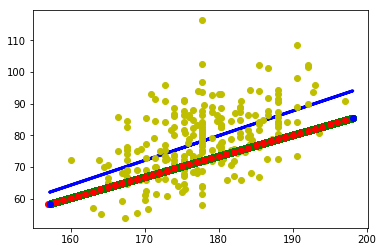Real DataAs you can see the regression line is some what close to the line of data that is generated. It is not perfect and there will be a lot of variability between the two datasets. But seeing that this is only based on two observations, (the min and max) the lines are pretty close. The intercept and slope are close enough to use the ones found from the two points only. So let us continue and make a fully synthetic deep learning dataset from two observations.

```# The slope of the line is b, and a is the intercept found from Sklenar linear model
# Simple Linear regressoin model Y = a + bX that will be the model for out MCMC
alpha =  -45.75941320293397 # Intercept
beta = [0.66259169] # Slope
X = np.array(large_hgt)
Y = np.array(large_pred)
print(len(X))
print(len(Y))

409000
409000

# Weight Histogram
hist = male.hist(column='wgt')
```

```#Normal distribution. mu is the mean, and sigma is the standard deviation.
# Seeing that the weight is normally distributed (basically) we can use that knowledge to generate new data via a normally
# Distrubuted method

#for random.normalvariate(mu, sigma)
std = np.std(X, axis=0)
real_std = np.std(male.wgt, axis=0)
print(std)
print(real_std)
11.806813005284504
10.491587167890629

temp_min_max = []
temp_min_max.append(male.wgt.max())
temp_min_max.append(male.wgt.min())
mean = np.mean(temp_min_max)
real_mean = np.mean(male.wgt)
print(mean)
print(real_mean)
85.15
78.14453441295547
```

Looking at the mean and standard deviation they are close enough for this example. Lets make a Million data points for our new dataset! That should be enough for any deep learning dataset.

```new_X = []
new_Y = []
for i in range(0,1000000):
index = randint(0, len(X) -1)
new_X.append(X[index])
new_Y.append(np.random.normal(mean,std))
plt.plot(new_X, new_Y, 'go',marker='^')
plt.plot(male.hgt, male.wgt, 'yo')
plt.plot(large_hgt, large_pred, 'go')
plt.plot(gen_height, prediction, 'ro')
plt.plot(old_min_hgt, old_min_wgt, 'bo')
plt.plot(old_max_hgt, old_max_wgt, 'bo')
plt.show()
```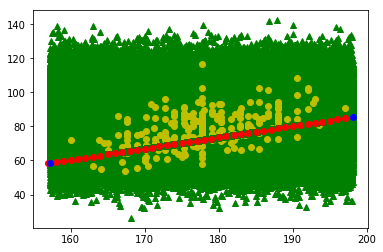A Million Data points!Well thats no good. Now to be fair, given a infinate number of samples, it is highly likely that at least for each point there would have been someone that mathces the height and weight on this chart, but that is like using a shotgun to fish. It is not as accurate and not really following the regression line of the real data which means that the dataset is not useful and cannot be used in a deep learning model as it wont learn anything.
So how can we fix this?
Let's perform some rejections by using a concept of banding. So if the observation falls outside the bands it won't get plotted. The bands themselves set up an upper and lower limit so that all predictions will have to fall within these limits. To form these limit expert knowledge of the observed phenomenon is needed especially for only two observations, luckily for us, we have more than two observations so we can define out limits based on the full real dataset.

```# Use upper and lower limits to reject samples
def make_sample(lower, upper, mean, std):
sample = np.random.normal(mean,std)
if lower < sample < upper:
return sample
else:
make_sample(lower, upper, mean, std)

# Define bands for each interval
# The more bands the finer the level of rejection
# Each item in the array is defined as
# [band lower, band upper, lower limit, upper limit]
band1 = [0, 155, 50, 70]
band2 = [156,160, 55, 70]
band3 = [161, 165, 56, 75]
band4 = [166, 170, 57, 80]
band5 = [171, 175, 60, 88]
band6 = [176, 180, 60, 94]
band7 = [181, 185, 60, 100]
band8 = [186, 190, 63, 105]
band9 = [191, 195, 64, 110]
band10 = [196, 299, 65, 110]
# Put all the bands into a single array for easy use
bands = []
bands.append(band1)
bands.append(band2)
bands.append(band3)
bands.append(band4)
bands.append(band5)
bands.append(band6)
bands.append(band7)
bands.append(band8)
bands.append(band9)
bands.append(band10)

new_X = []
new_Y = []
for i in range(0, 1000000):
index = randint(0, len(X) -1)
for band in bands:
if band <= X[index] <= band:
new_X.append(X[index])
new_Y.append(make_sample(band, band, mean, std))

plt.plot(new_X, new_Y, 'go',marker='^')
plt.plot(male.hgt, male.wgt, 'yo')
plt.plot(large_hgt, large_pred, 'go')
plt.plot(gen_height, prediction, 'ro')
plt.plot(old_min_hgt, old_min_wgt, 'bo')
plt.plot(old_max_hgt, old_max_wgt, 'bo')
plt.show()
```

Which gives us this!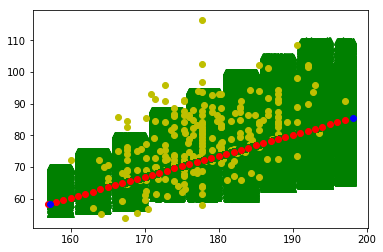Banded Data There are still a million points, but some my be repeated. But, the general flow is far more similar to the real data which is perfect now for training a deep learning model.

### Conclusion

From this blog, we saw how to use only two observations, the minimum and maximum, and how to create a fully synthetic dataset that can be used for deep learning.
The main idea when building a fully synthetic dataset is to ensure it is statistically and logically similar to that of the observed/real dataset. This gives the benefit of creating a large training dataset and then using the real data as a testing set. This can give very good results when creating a deep learning model as you won't have to train the model on the very limited (and precious) real data that can be very difficult to capture or collect.

This approach can be improved significantly, especially in the banding section. By adding a larger number of bands, smoothing out the lower and upper limits, and even using more complex algorithms like a random walk can improve the final results. But, this method still needs to be vetted before use in different models and/or real-world applications. The next step would be to model more independent variables, other phenomenons, and improve the generation steps.

### References:

Heinz G, Peterson LJ, Johnson RW, Kerk CJ. 2003. Exploring Relationships in Body Dimensions. Journal of Statistics Education 11(2).